Next: Ensemble learning for hidden Up: Hidden Markov models Previous: Continuous observations   Contents

## Learning algorithms

Rabiner  lists three basic problems for HMMs:

1. Given the observation sequence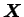and the model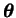, how can the probability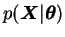be computed efficiently?
2. Given the observation sequenceand the model, how can the corresponding optimal state sequence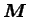be estimated?
3. Given the observation sequence, how can the model parametersbe optimised to better describe the observations?

Problem 1 is not a learning problem but rather one needed in evaluating the model. Its difficulty comes from the fact that one needs to calculate the sum over all the possible state sequences. This can be diverted by calculating the probabilities recursively with respect to the length of the observation sequence. This operation forms one half of the forward-backward algorithm and it is very typical for HMM calculations. The algorithm uses an auxiliary variable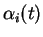which is defined as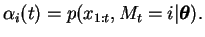(4.8)

Here we have used the notation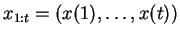.

The algorithm to evaluateproceeds as follows:

1. Initialisation: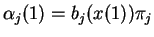2. Iteration: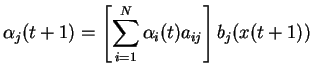3. Termination: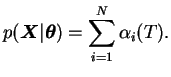The solution of Problem 2 is much more difficult. Rabiner uses classical statistics and interprets it as a maximum likelihood estimation problem for the state sequence, the solution of which is given by the Viterbi algorithm. A Bayesian solution to the problem can be found with a simple modification of the solution of the next problem which essentially uses the posterior of the hidden states.

Rabiner's statement of Problem 3 is more precise than ours and asks for the maximum likelihood solution optimisingwith respect to. This problem is solved by the Baum-Welch algorithm which is an application of the EM algorithm to the problem.

The Baum-Welch algorithm uses the complete forward-backward procedure with a backward pass to compute. This can be done very much like the forward pass:

1. Initialisation: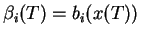2. Iteration: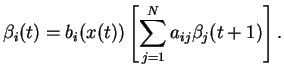The M-step of Baum-Welch algorithm can be expressed in terms of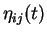, the posterior probability that there was a transition between state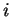and state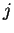at time step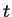givenand. This probability can be easily calculated with forward and backward variables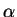and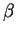: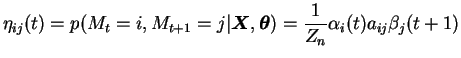(4.9)

where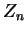is a normalising constant such that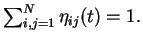Then the M-step for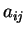is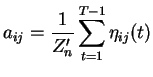(4.10)

where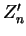is another constant needed to make the transition probabilities normalised. There are similar update formulas for other parameters. The algorithm can also easily be extended to take into account the possible priors of different variables .

SubsectionsNext: Ensemble learning for hidden Up: Hidden Markov models Previous: Continuous observations   Contents
Antti Honkela 2001-05-30